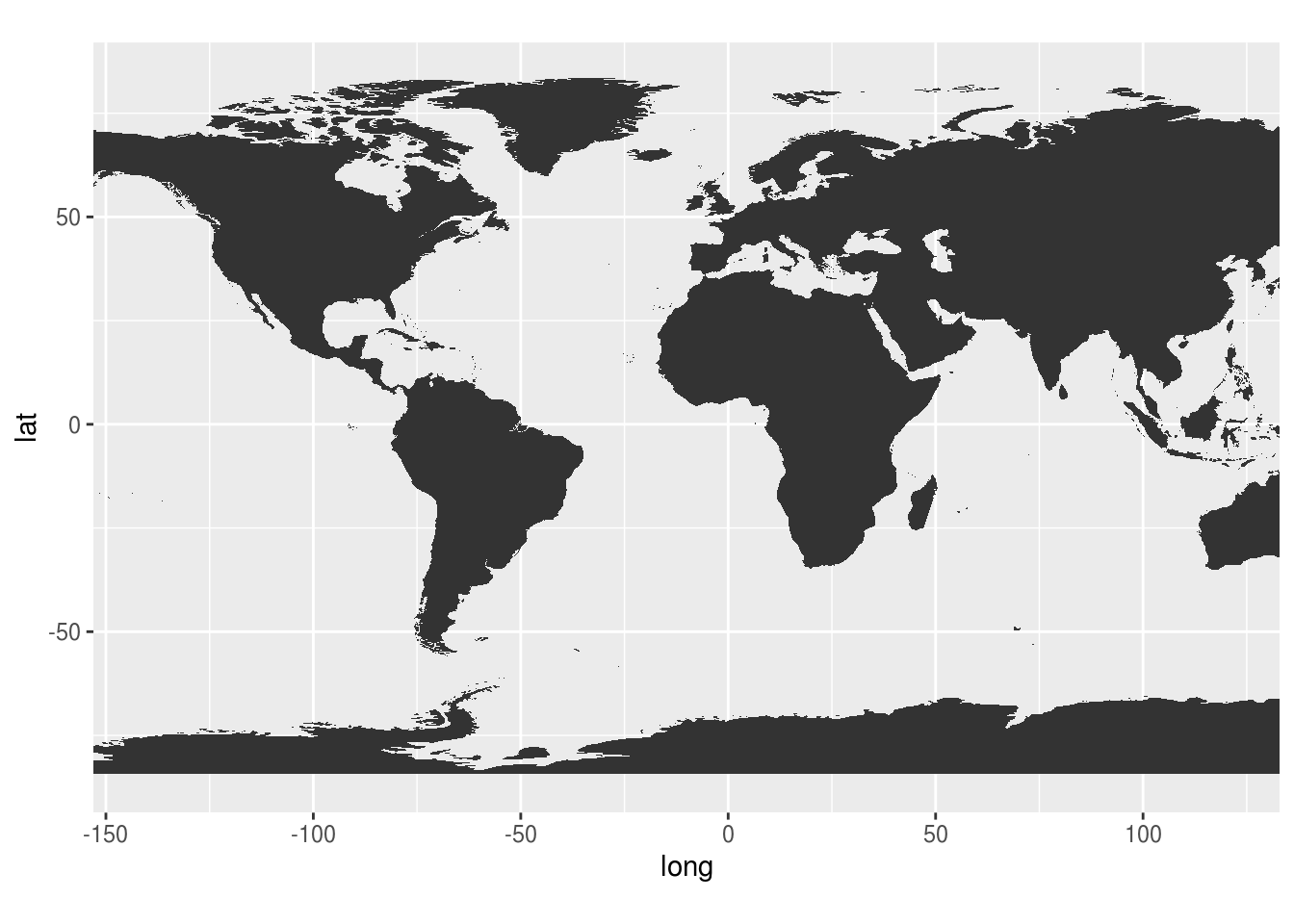# 3 绘制地图

require(ggplot2)
p <- ggplot(map_data("world"),
aes(long,lat,group=group)) +
geom_polygon()
p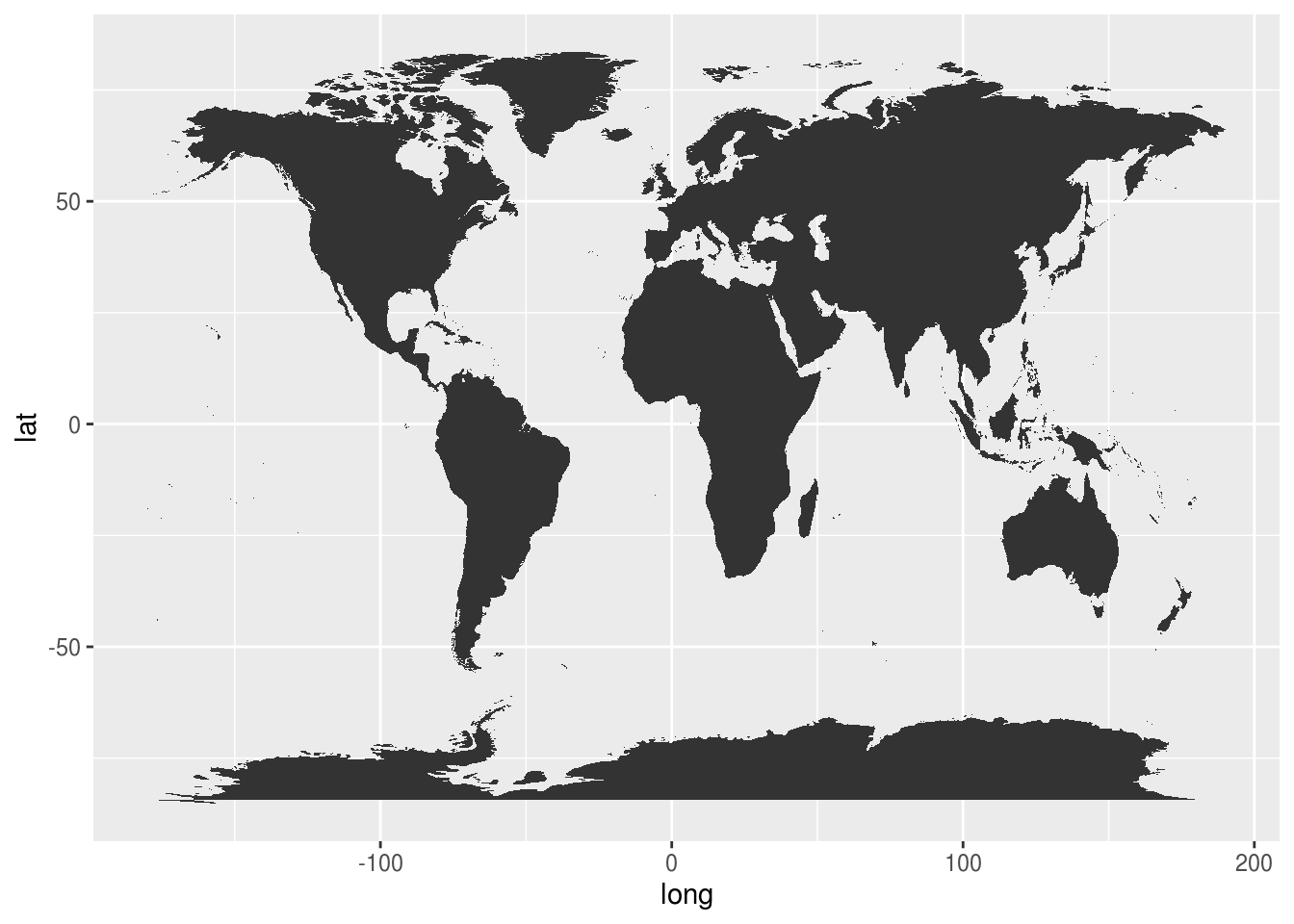lr <- c(-140, 120)
p + coord_map(xlim = lr)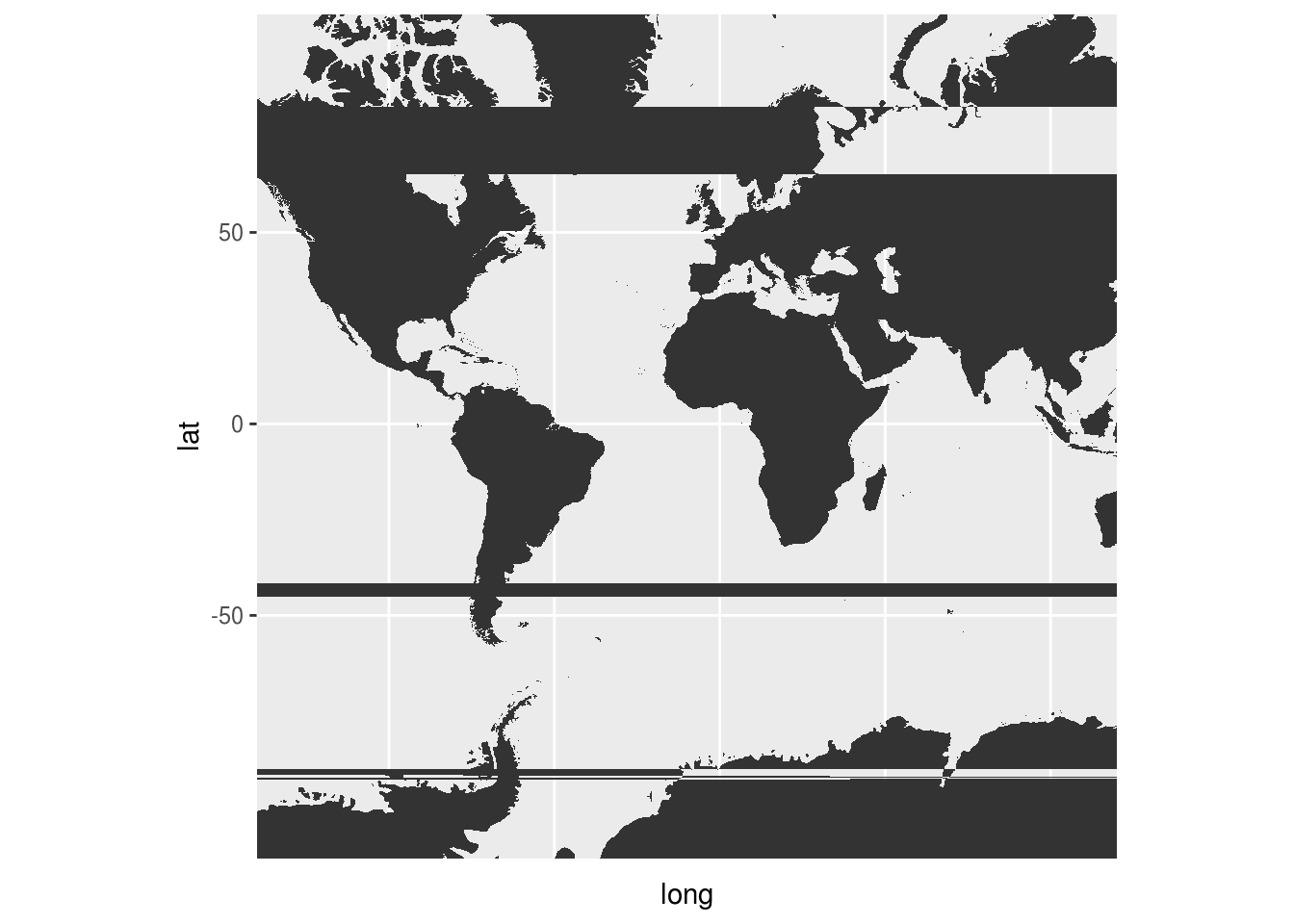SO 上类似的讨论给出的解答只在 xlim=c(-180,180) 适用。换个小范围就不行了。

ggplot2 官方说明书 章节末尾给出的示例图就是乱的，居然没有任何说明：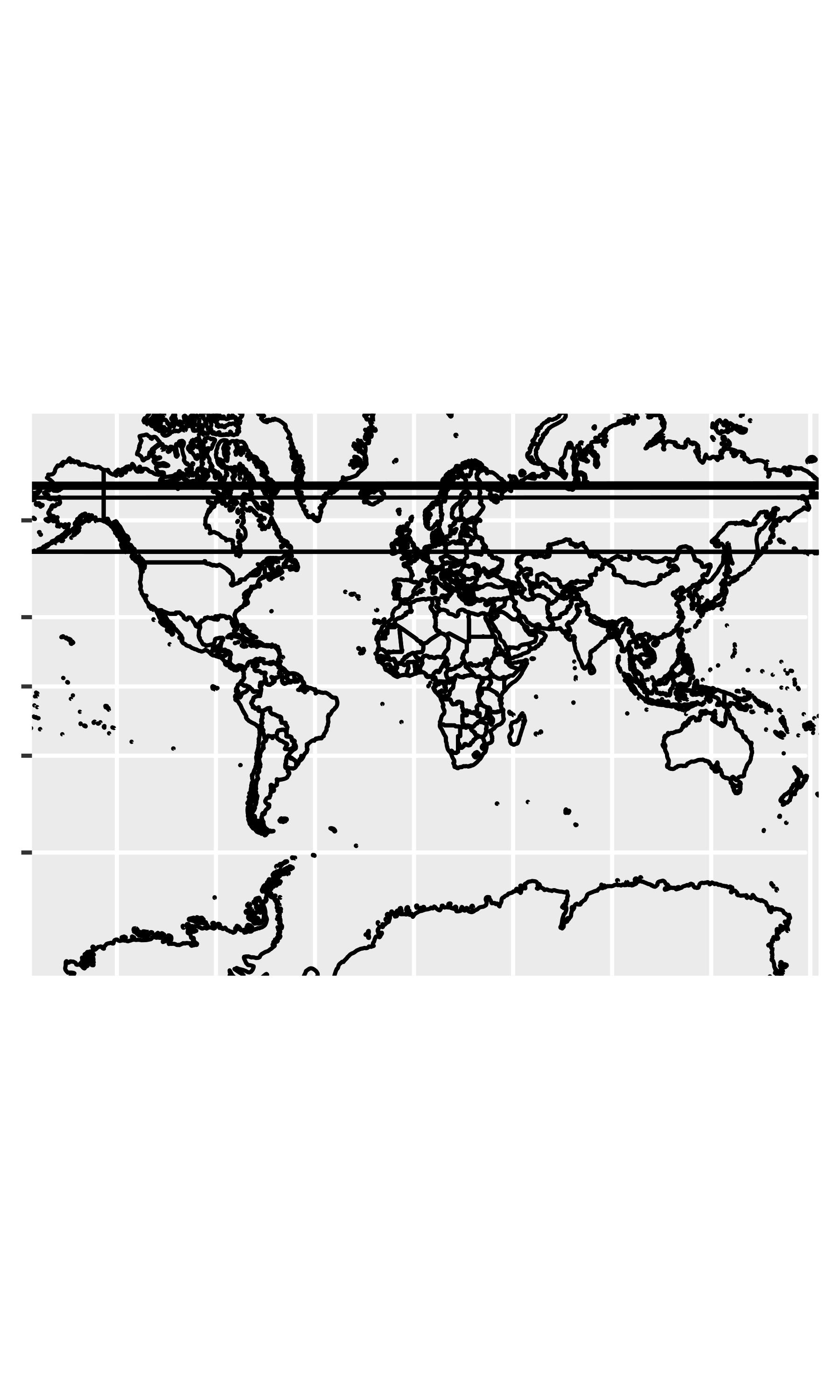N 版

maps::map("world",
fill=TRUE,
col="white",
bg="lightblue",
xlim = c(-140, 120))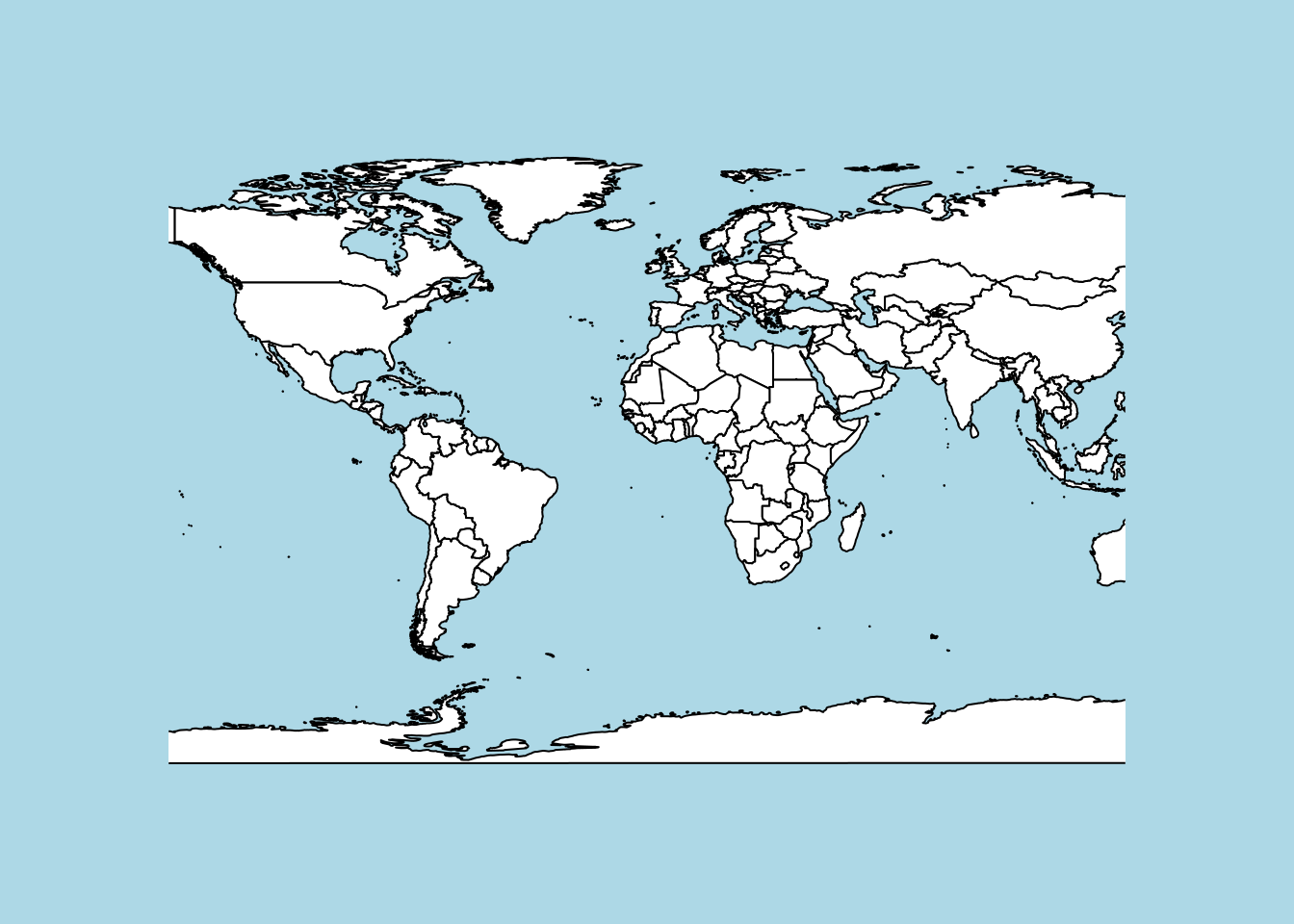T 版

coord_map() 函数里用 projection 更换投影坐标系，用 orientation 选择视角。

p +
coord_map(xlim = lr,
orientation = c(90, 0, 0)) +
scale_y_continuous(breaks = -9:9 * 10)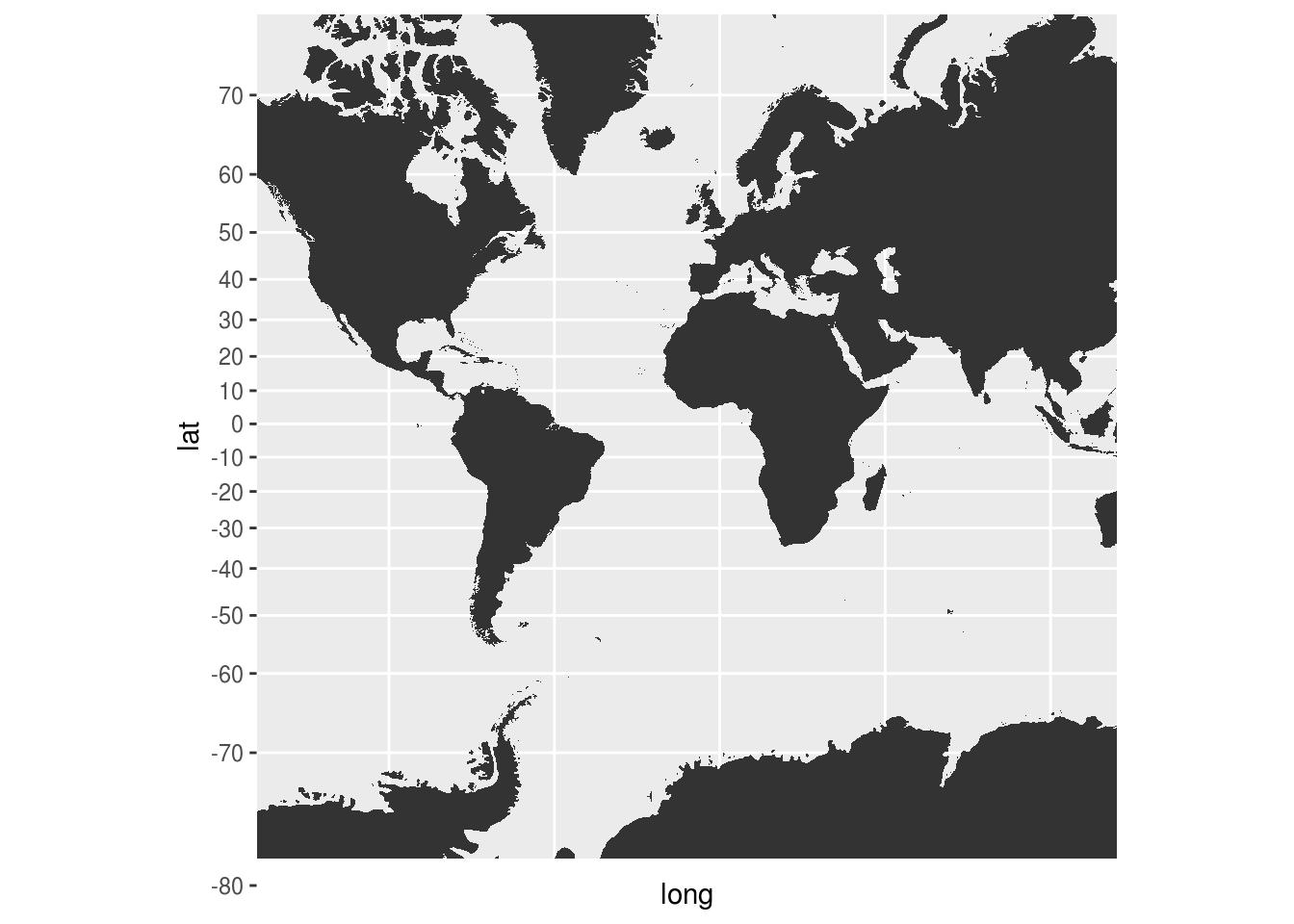p + coord_map("orthographic", xlim = lr)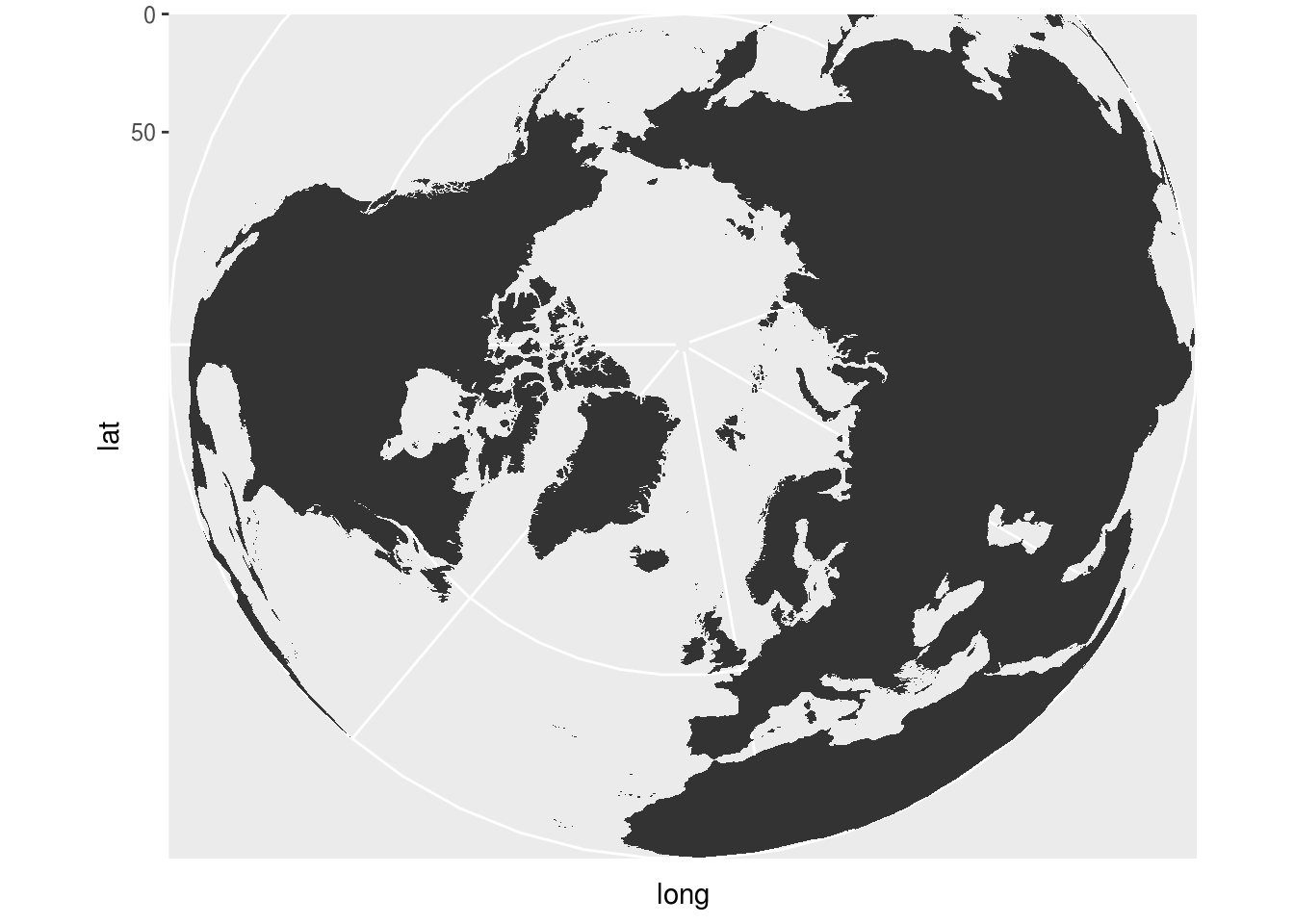p + coord_quickmap(xlim = lr)